Home Catalog

### Graph Theory

Chapter 1 - Mathematical Models

Chapter 2 - Elementary Concepts of Graph Theory

### Chapter 1 - Mathematical Models

1.1 Nonmathematical Models

1.2 Mathematical Models

1.3 Graphs

1.4 Graphs as Mathematical Models

1.5 Directed Graphs as Mathematical Models

1.6 Networks as Mathematical Models

### Chapter 2 - Elementary Concepts of Graph Theory

2.1 The Degree of a Vertex

2.2 Isomorphic Graphs

2.3 Connected Graphs

2.4 Cut-Vertices and Bridges

### 1.1 Nonmathematical Models

Probably the best way to learn what “mathematical models” are is to look at examples. This is what we shall do in this chapter. We begin, however, by retreating one step to the word “model,” since models need not be mathematical. We shall see that the difficulties involved in “constructing” mathematical models may be very similar to the steps in building nonmathematical models.

What exactly does the word “model” mean? Let us consider some uses of this word. Suppose you, the reader, and your husband (perhaps you have a different “model” of a reader!) have received in the mail a brochure which advertises a new land development near your city, including private houses, apartment complexes, and shopping areas. The brochure shows a map of this area. Curved and straight lines represent roads, rectangles represent houses, and other diagrams represent other aspects of this new development. You know, of course, that the map and what it displays is not the actual land development. It is only a model of the development.

You have been considering moving from your current apartment, so, with the aid of the map, you and your husband drive to the apartment complex. This drive turns out to be more difficult than anticipated since all the roads leading into the area are dirt roads and very bumpy. (The map didn’t mention that!) You arrive at the office of the apartment complex, and in the middle of the room is a large table displaying a miniature model of the entire complex. This allows you to see the location of the apartment buildings as well as the office, the swimming pool, the roads, and the children’s play area. Several things which are important to you (such as the location of laundry facilities and carports) are not shown in the model, so you ask about these.

You are interested in this new apartment complex and you would like to see what a typical 2-bedroom apartment looks like. So you are directed to a model apartment. Although all the apartments available are unfurnished, the model apartment is furnished to help you determine its appearance once you have moved in. However, the model apartment is a bit misleading, for it has been elegantly decorated by a local furniture store while your furniture is perhaps quite ordinary at best.

We have now seen three examples of models. In each case, the model is a representation of something else. Whether the model gives an accurate enough picture of the real entity depends entirely on which features are important to you.

How else is the word “model” used? Perhaps you (a different reader) think of an attractive young woman modeling a swimsuit. In this case, the manufacturer or a department store is trying to sell swimsuits, and rather than displaying them at a counter, they are having a model give you an idea of how the suit would look on your wife (or your sister) if you were to buy it. In this case, the model may not give you a very accurate picture of what the swimsuit will look like on the person for whom it is purchased; on the other hand, you may not care.

Another common use of the word “model” is in “model car” or “model airplane.” Perhaps you’d like to build a model of a 1956 Thunderbird. There are model kits available for this purpose, but these may not be satisfactory if you would like your model to illustrate the dashboard. There must be some limitations on the detail of your model, or otherwise, the only possibility is to purchase your own 1956 Thunderbird.

Intuitively, then, a model is something which represents something else. It may be smaller, larger, or approximately the same size as the thing it represents. It illustrates certain key features (but not all features) of the real thing. What features it possesses depends completely on the construction of the model. Ideally, a model should possess certain predetermined characteristics. Whether such a model can be built is often the crucial problem.

### 1.2 Mathematical Models

In a mathematical model, we represent or identify a real-life situation or problem with a mathematical system. One of the best-known examples of this representation is plane Euclidean geometry or plane trigonometry, which gives useful results for describing small regions, such as measuring distances. For example, the map of a state would be very useful for determining distances between towns and cities in the state, but in many instances a map of the world would not be as helpful as a globe, say, for calculating distances between certain cities. To indicate how varied mathematical models may be, we present several examples.

Example 1.1
For investment purposes, you have been building apartment houses the past three years. In particular, three years ago you built a 4-apartment building for $100,000. Two years ago, you raised a 6-apartment building for$140,000, and last year you completed an 8-apartment building for $180,000. You are now considering your construction plans for the current year. What kind of mathematical model would represent this situation? You might observe that in each case the cost C of the apartment building equals the sum of$20,000 and the product of the number n of apartments and $20,000, that is, $$C=20,000+20,000n$$ for n = 4, 6, 8. We might use this formula, then, to model the cost of apartment buildings. Example 1.2 You left a jug of wine sitting in your car and its temperature is 70°F when you remove it. You place the jug in your refrigerator, where the temperature is 35°. After 30 minutes you observe that the temperature of the wine has dropped to 60°. What mathematical model would represent this situation? Here we might refer to a law of physics which, in this case, states that the rate of change of the temperature T of the wine is proportional to the difference between the temperatures of the wine and of the refrigerator: $\frac{dT}{dt}=k\left(T−35\right)$ where k is the constant of proportionality and t denotes time. In order to arrive at an expression for T, it would be necessary to solve this differential equation. Example 1.3 You are a member of an organization which has just purchased a new major league baseball franchise: the New Orleans Shrimps. Each of the existing major league teams has agreed to leave unprotected four of their players, and you have the option of purchasing the contract of any of these unprotected players at$75,000 each, provided no more than two players are taken from any one team. You have the records of each of these players. Naturally, you would like to obtain the best players available for your team.What mathematical model would represent this situation? First, you decide to rank pitchers according to their earned-run averages. Then, for non-pitchers, a more complicated formula is adopted. For each player’s preceding season, let h be the number of hits, H the number of home runs, r the number of runs scored, R the number of runs batted in, w the number of times the player had the winning hit, f his fielding average, and b his batting average. Then the player’s proficiency P is given by

$$P=h+5H+r+2R+10w+1000f+1000b$$

The non-pitchers are then ranked according to their player proficiencies.

Example 1.4

This situation can be represented by the diagram of Figure 1.1, where the “states” S0, S1, S2, and S3 are as follows:

• S0: You are an undergraduate (initial state).
• S1: You look for a job in the business world.
• S2: You travel with your friends.
• S3: You go to graduate school.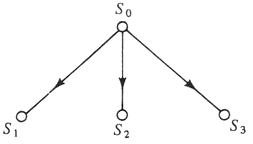You now know what the alternatives are, but what decision should you make? Such a decision requires more information. After thinking this over, you decide the following criteria are most important to you:

• How interesting this activity will be.
• How this will eventually affect your getting an interesting and challenging job.
• How this affects your meeting young men.
• How this will affect your immediate financial status.
• How much free time this will give you.

You decide to assign 1, 2, or 3 points to each of these factors in each alternative state. The values assigned are:

 S1 S2 S3 interesting activity 2 3 2 eventual job 2 1 3 meeting young men 2 2 3 financial status 3 1 1 free time 1 3 2 — — — 10 10 11

Therefore you decide to go to graduate school. Now where do you apply?—that’s another decision.

Example 1.5
You own a rather exclusive golf course in the country, and you are trying to decide what you should charge for a round of golf. You decide to try an experiment. You charge $11.75 one day and 50 golfers play that day. When you charge$11.00, a total of 100 people pay to play golf. When you charge \$9.75, the number of golfers totals 150. What mathematical model would represent this situation? First we observe that for x = 50, 100, 150, the price p which is being charged is given by

$$p=12−0.0001x^2$$

Hence, we might choose this formula as our model.

### 1.3 Graphs

Before proceeding further with illustrations of mathematical models, we pause to introduce the concept of a “graph.” As the title of this book indicates, we shall encounter this concept many times. A graph G is a finite nonempty set V together with an irreflexive, symmetric relation R on V. Since R is symmetric, for each ordered pair (u, v) ∈ R, the pair (v, u) also belongs to R. We denote by E the set of symmetric pairs in R. For example, a graph G may be defined by the set

V = {v1, v2, v3, v4}

together with the relation

R = {(v1,v2), (v1, v3), (v2,v1), (v2,v3), (v3,v1), (v3,v2), (v3,v4), (v4,v3)}.

In this case,

E = {{(v1,v2), (v2,v1)}, {(v1,v3), (v3,v1)}, {(v2,v3), (v3,v2)}, {(v3,v4), (v4,v3)}}.

In a graph G, we refer to V as the vertex set, each element of V being called a vertex (the plural is “vertices ”). The number of vertices in G is called the order of G. Each element of E (that is, each set consisting of two symmetric ordered pairs from R) is called an edge, and E itself is called the edge set of G. The number of edges in G is called the size of G. Hence, |V| = order of G and |E| = size of G.

If G is a graph defined in terms of a vertex set V and a relation R on V, then (u, v) ∈ R implies (v, u) ∈ R. Hence, {(u, v), (v, u)} is an edge of G. It is convenient to denote such an edge by uv (or, equivalently, vu). The edge set E completely determines the relation R; indeed, it is customary to describe a graph in terms of its vertex set and edge set. The graph G illustrated above could then be defined in terms of the sets V = {v1, v2, v3, v4} and E = {v1v2, v1v3, v2v3, v3v4}. Therefore, the order of G is four, as is its size.

Occasionally it is desirable to denote the vertex set and edge set of a graph G by V(G) and E(G), respectively. This is particularly useful when there are two or more graphs under consideration.

Since the empty subset of V × V is an irreflexive and symmetric relation on V, it follows that the edge set of a graph may be empty, i.e., a graph may have no edges. Of course, by definition, every graph has vertices.

In dealing with graphs, it is often convenient to represent them by means of diagrams. In such a representation, we indicate the vertices by points or small circles, and we represent the edges by line segments or curves joining the two appropriate points. The line segments or curves are drawn so that they pass through no point other than the two points they join. Diagrams of the graph G previously described are given in Figure 1.2. The first diagram uses only straight line segments, while the second diagram employs curved lines. Although the two diagrams look quite different, they contain exactly the same vertices and the same edges, and so they describe the same graph. Notice that in the second diagram, the line segments representing the edges v1v2 and v3v4 intersect. This is quite permissible (in fact, it may be unavoidable), but you should not confuse this point of intersection with a vertex. As mentioned earlier, for this example, there are four vertices.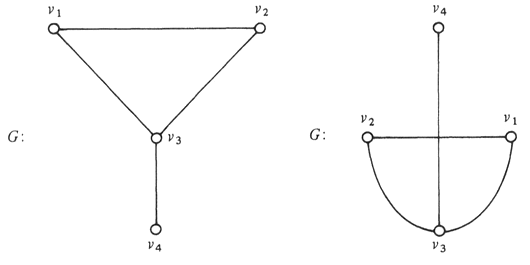Since a diagram of a graph (such as the diagram shown in Figure 1.2) completely describes the graph, it is customary and convenient to refer to the diagram of a graph G as G itself. A few elementary definitions are now in order. (Many of these are inspired by the geometric aspect of graphs.)

If e = uv ∈ E(G) (i.e., if uv is an edge of a graph G), then we say e joins the vertices u and v. Two vertices u and v are adjacent in a graph G if uv ∈ E(G). We say that u and v are adjacent to or adjacent with each other. If uv ∉ E(G), then u and v are nonadjacent vertices. If e = uv ∈ E(G), then u and v are each incident to or incident with e. If uv and uw are distinct edges of a graph G (i.e., v ≠ w), then uv and uw are adjacent edges. Hence, in the graph G of Figure 1.2, v1 and v3 are adjacent, but v1, and v4 are not adjacent. The vertex v3 is incident to the edge v2v3, but v4 is not incident to v2v3. The edges v1v3 and v3v4 are adjacent, but v1v2 and v3v4 are not adjacent.

### 1.4 Graphs as Mathematical Models

The construction of mathematical models may take many forms and may involve many areas of mathematics. One area of mathematics particularly well-suited to model building is graph theory. In this section we present examples of situations and describe the appropriate graphs that serve as mathematical models. At this point, we make no attempt to consider detailed problems. We shall delay discussion of problems until Chapter 3.

Example 1.6
A grade-school teacher wishes to make a seating chart for her class. How she constructs the seating chart may depend on which students are friends of each other. The class can be “pictured” by means of a graph, where the vertices represent the students and friendship between two students is indicated by an edge between the appropriate vertices.

Example 1.7
Several army platoons are deployed in various locations in preparation for a battle. Communication is handled by battery-powered telephone. Two platoons can communicate directly with each other if they are sufficiently close. A model of this situation would be a graph where the vertices represent the platoons and direct communication between two platoons is represented by an edge between the two appropriate vertices.

Example 1.8
A number of islands are located in the Pacific Ocean off the California coast. Suppose a line of ferryboats operates from the mainland to certain of the islands. Suppose further that boats travel between several islands as well. This situation can be represented by means of a graph, where the vertices denote the islands and the mainland (one vertex for each island and one for the mainland). Two vertices are joined by an edge if you can travel by boat directly between the land areas.

### 1.5 Directed Graphs as Mathematical Models

A directed graph D (often called a digraph) is a finite nonempty set V together with an irreflexive relation R on V. As with graphs, the elements of V are called vertices. Each ordered pair in R is referred to as a directed edge or arc (the word “edge” is not used in digraphs). For consistency with the notation introduced for graphs, we shall denote the relation by E rather than by R when dealing with digraphs.

Since the defining relation of a digraph D need not be symmetric, it follows that if (u, v) is an arc of D, then (v, u) need not be an arc of D. This situation would be indicated in a diagram of D by drawing a line segment or curve between the points representing u and v and inserting an arrowhead that “directs” the line segment from u to v. Should both (u, v) and (v, u) be arcs of D, then, ordinarily, we would draw two curves (which do not cross) between u and v, and place an arrowhead on each curve in opposite directions.

If we let V1= {v1, v2, v3, v4} and E1= {(v1, v2), (v2, v3), (v3, v2)}, then we have described a digraph, say D1. The digraph D1 can be indicated pictorially, as in Figure 1.3. Again we shall follow the custom of referring to a diagram of a digraph as the digraph itself.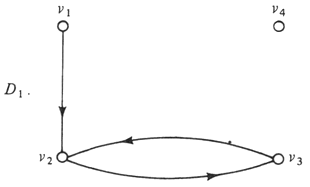It may happen that the relation defining a digraph D is symmetric. We refer to such digraphs as symmetric digraphs. Of course, symmetric digraphs are then graphs. The only real difference between a symmetric digraph and a graph is how they are represented pictorially. For example, Figure 1.4 shows a symmetric digraph D and its graphical counterpart G.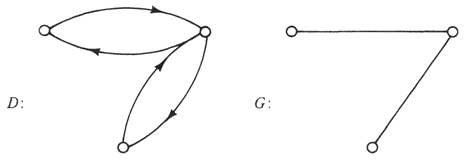There are certain situations for which digraphs yield a more acceptable mathematical model than graphs can provide. We consider two examples.

Example 1.9
A large New York business firm has a rather complex structure. We can represent this structure by a digraph D. Namely, we identify a vertex of D with each individual working in the firm. Then we draw an arc from vertex u to vertex v if the individual associated with v is a subordinate of the individual associated with u.

Example 1.10
A city has several one-way streets as well as two-way streets. The traffic pattern of the city may be indicated by means of a digraph. For example, we could represent the street intersections by vertices, and introduce an arc from u to v if it is possible to drive legally from the intersection associated with u to the intersection associated with v without passing through any other intersection.

### 1.6 Networks as Mathematical Models

Just as there are instances when digraphs are more suitable than graphs as mathematical models for certain situations, there are occasions when neither graphs nor digraphs are entirely appropriate as mathematical models, although graphs or digraphs appear to be involved. In this section we consider some other alternatives.

By a network we mean a graph or digraph together with a function which maps the edge set into the set of real numbers. (The word “network” is used because of its connection with electrical networks.) A network resulting from a graph is called an undirected network, while a network resulting from a digraph is called a directed network. Examples of each are shown in Figure 1.5.A signed graph S is an undirected network whose functional values are + 1 or −1. Since a positive or negative sign is attached to every edge of S, it is natural to refer to each edge of a signed graph as a positive edge or negative edge. For example, if

V = {v1, v2, v3},
E = {v1v2, v1v3, v2v3},

and

f = {(v1v2, + 1), (v1v3, −1), (v2v3, −1)},

then the resulting signed graph can be represented in one of two ways, as shown in Figure 1.6.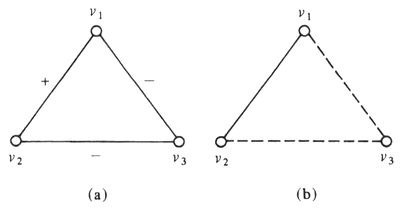Example 1.11
A neighborhood consists of several families. Two families may be friendly toward each other, unfriendly, or may be indifferent toward (or may not even be acquainted with) each other. This situation can be represented by a signed graph S, where the vertices are joined by a positive edge if the corresponding families are friendly, by a negative edge if the corresponding families are unfriendly, and by no edge otherwise.

Undirected networks whose functional values are positive integers often serve as mathematical models. There are two common ways to represent such undirected networks. For example, if

V = {v1, v2, v3},
E = {v1v2, v1v3, v2v3},

and

f = {(v1v2, 2), (v1v3, 1), (v2v3, 3)},

then the resulting undirected network can be represented as shown in Figure 1.7(a) or Figure 1.7(b).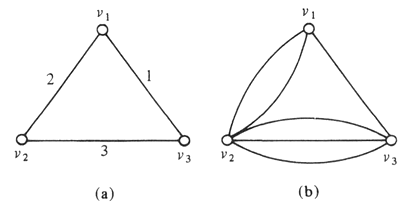If such an undirected network is represented as a set of points in the plane and the points are joined by an integral number of curves or line segments [as in Figure 1.7(b)], then the network is called a multigraph. Let M be a multigraph with edge set E and associated function f. If uv ∈ E and f(uv) = n, where n is a positive integer, then we say u and v are joined by n edges, and we refer to these edges as multiple edges.

Example 1.12
Let v1, v2, and v3 be three villages, and suppose a road runs between every two villages. The quality of the roads and the distances between villages make the walking times between the villages as follows:

• between v1 and v2, two days;
• between v1 and v3, one day;
• between v2 and v3, three days.

This situation can be represented as in Figure 1.7(a).

Example 1.13
Suppose v1, v2, and v3 are three villages, and suppose there are two roads between v1 and v2, one road between v1 and v3, and three roads between v2 and v3. This situation can be represented as in Figure 1.7(b).

In all the relations we have considered in this chapter, we have assumed irreflexivity. It is quite possible that in the situation under discussion, the relation is not irreflexive. In this’case, we refer to the ordered pair (u, u) as a loop. If we remove the restriction “irreflexive” in the definition of graph, we call the result a loop-graph. Loopdigraphs, loop-networks, and loop-multigraphs are defined analogously. Loop-multigraphs are also called pseudographs.

Let

V = {v1, v2, v3, v4}

and

E = {(v1, v2), (v2, v3), (v3, v2), (v3, v3), (v4, v4)}.

This is a loop-digraph, which could be drawn as indicated in Figure 1.8.### 2.1 The Degree of a Vertex

We have already introduced two numbers associated with a graph G, namely the order and the size. Now we define a collection of numbers associated with G. Let v be a vertex of G. The number of edges of G incident with v is called the degree of v in G. The degree of v is denoted by degG v, or simply deg v if the graph is clear by context. For the graph G of Figure 2.1, deg v1 = 1, deg v2 = 2, deg v3 = 3, deg v4 = 2, and deg v5 = 0.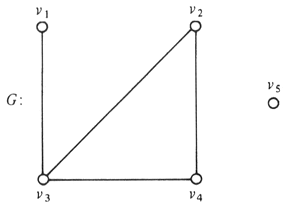By a (p, q) graph we mean a graph having order p and size q. The graph G of Figure 2.1 is a (5, 4) graph. We might observe that for this graph, the sum of the degrees of its vertices is 8, which, in this case, equals 2q. This is no mere coincidence, as we now show.

Theorem 2.1
For any graph G, the sum of the degrees of the vertices of G equals twice the number of edges of G. Symbolically, if G is a (p, q) graph with vertices v1, v2, . . . , vp, then

Σi=1p deg vi = 2q

Proof
When summing the degrees of the vertices of a graph G, we count each edge of G twice, once for each of the two vertices incident with the edge. ▋

A vertex is called even or odd according to whether its degree is even or odd. The graph G of Figure 2.1 has two odd vertices and three even vertices. The following result is a consequence (or corollary) of Theorem 2.1.

Theorem 2.2
Every graph contains an even number of odd vertices.

Proof
Let G be a graph. If G contains no odd vertices, then the result follows immediately. Suppose that G contains k odd vertices; denote them by v1, v2, . . . , vk. If G contains even vertices as well, then denote these by u1, u2, . . . , un. By Theorem 2.1,

(deg v1 + deg v2 + . . . + deg vk) + (deg u1 + deg u2 + . . . + deg un) = 2q,

where q is the number of edges in G. Since each of the numbers deg u1, deg u2, . . . , deg un is even, (deg u1 + deg u2 + . . . + deg un) is even, so we have

(deg v1 + deg v2 + . . . + deg vk) = 2q - (deg u1 + deg u2 + . . . + deg un)   is even.

However, each of the numbers deg v1, deg v2, . . . , deg vk is odd. Therefore, k must be even; that is, G has an even number of odd vertices. If G has no even vertices, then we have (deg v1 + deg v2 + . . . + deg uk) = 2q, from which we again conclude that k is even. ▋

If every vertex of a graph G has the same degree r, we say that G is regular of degree r or is r-regular. A graph is complete if every two of its vertices are adjacent. A complete graph of order p is (p − 1)-regular and is denoted by Kp. Five complete graphs are shown in Figure 2.2.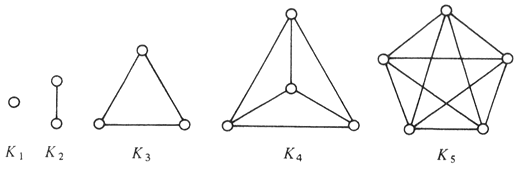### 2.2 Isomorphic Graphs

In every area of mathematics, it is important to know whether two objects under investigation are the same (in some sense) or are different. For example, the numbers 2 and 6/3 are considered to be the same, or equal, but they are certainly not identical. We now wish to determine what conditions must hold for two graphs to be “equal.” The importance of knowing this equality lies in the fact that if G1 and G2 are two equal graphs which are models of two situations, then there is something basically similar about the two situations.

Intuitively, two graphs G1 and G2 are the same if it is possible to redraw one of them, say G2, so it appears identical to G1. For example, the graphs G1 and G2 of Figure 2.4 have this property.

We refer to two equal graphs as “isomorphic graphs.” We now give a more formal definition of this concept. Let G1 and G2 be two graphs. By an isomorphism from G1 to G2 we mean a one-to-one mapping φ : V(G1) → V(G2) from V(G1) onto V(G2) such that two vertices u1 and v1 are adjacent in G1 if and only if the vertices φ(u1) and φ(v1) are adjacent in G2. We then say that G1 and G2 are isomorphic if an isomorphism exists from G1 to G2. If φ is an isomorphism from G1 to G2, then the inverse mapping φ−1 (see Exercise A.25) from V(G2) to V(G1) also satisfies the definition of isomorphism. If G1 and G2 are isomorphic graphs, we can say that G1 is isomorphic to G2 and that G2 is isomorphic to G1.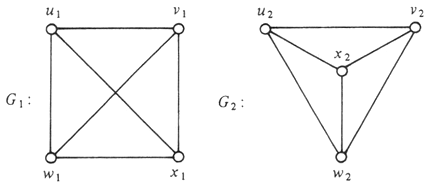An important property of isomorphism is contained in the following theorem.

Theorem 2.3
The relation “is isomorphic to” is an equivalence relation on the set of all graphs.

Proof
The fact that the relation “is isomorphic to” is reflexive follows immediately. We need only observe that if G is a graph and the mapping φ : V(G) → V(G) is defined by φ(v) = v for all v ∈ V(G), then φ is an isomorphism from G to G, i.e., G is isomorphic to G.

Suppose G1 is isomorphic to G2; that is, suppose φ is an isomorphism from G1 to G2. Define the inverse−mapping φ−1: V(G2) → V(G1) by φ−1(v2) = v1 if φ(v1) = v2. By Exercise A.25, φ−1 is a one-to-one mapping from V(G2) onto V(G1). Suppose u2, v2 ∈ V(G2), and φ−1 (u2) = u1 and φ−1(v2) = v1. Then φ(u1) = u2 and φ(v1) = v2. From these last equalities, u2 and v2 are adjacent if and only if φ(u1) and φ(v1) are adjacent, and since G1 is isomorphic to G2, φ(u1) and φ(v1) are adjacent if and only if u1 = φ−1(u2) and v1 = φ−1(v2) are adjacent. Therefore, u2 and v2 are adjacent if and only if φ−1(u2) and φ−1(v2) are adjacent. This shows that G2 is isomorphic to G1, i.e. “is isomorphic to” is a symmetric relation.

We still must show that the relation is transitive. Suppose that G1 is isomorphic to G2 and that G2 is isomorphic to G3. Hence there exist isomorphisms α: V(G1) → V(G2) and β: V(G2) → V(G3). Consider the composite mapping β ⃘ α. By Theorems A.4 and A.5 (page 254), β ⃘ α is a one-to-one mapping from V(G1) onto V(G3). Let u1, v1 ∈ V(G1). Suppose that α(u1) = u2 and α(v1) = v2, and that β(u2) = u3 and β(v2) = v3. Since α and β are isomorphisms, u1 and v1 are adjacent if and only if α(u1) = u2 and α(v1) = v2 are adjacent; and u2 and v2 are adjacent if and only if β(u2) = u3 and β(v2) = v3 are adjacent. Thus, u1 and v1 are adjacent if and only if u3 = (β ⃘ α)(u1) and v3 = (β ⃘ α)(v1) are adjacent. This completes the proof that β ⃘ α is an isomorphism.

Hence, G1 is isomorphic to G3. ▋

By Theorem A.2, it follows that the equivalence relation “is isomorphic to” partitions the set of all graphs into equivalence classes. Hence, two graphs which belong to the same equivalence class are isomorphic, while two graphs belonging to different equivalence classes are not isomorphic (that is, they are considered different graphs).

If G1 and G2 are isomorphic graphs, then, by definition, there exists a one-to-one mapping φ from V(G1) onto V(G2). This implies that V(G1) and V(G2) have the same number of elements; that is, G1 and G2 have the same order. Let u1 and v1 be two vertices of G1, and suppose that φ(u1) = u2 and φ(v1) = v2. Then u1 and v1 are adjacent in G1 if and only if u2 and v2 are adjacent in G2, or in other words, u1v1 is an edge of G1 if and only if u2v2 is an edge of G2. This implies that G1 and G2 have the same size. However, if two graphs have the same order and the same size, it does not necessarily follow that the graphs are isomorphic. For example, the two graphs of Figure 2.5 have order six and size nine, but they are not isomorphic.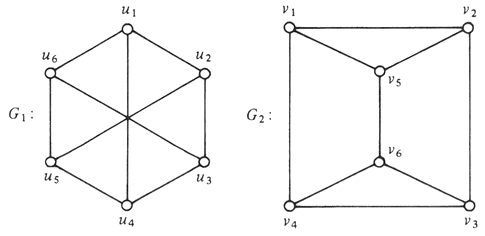It may seem a difficult problem to show that the graphs G1 and G2 of Figure 2.5 are not isomorphic, for evidently, we must verify that every one-to-one mapping from V(G1) onto V(G2) [or from V(G2) to V(G1)] fails to be an isomorphism. However, we can simplify the problem immensely by making some pertinent observations. In the case of the graphs G1 and G2 of Figure 2.5, consider any one-to-one mapping φ from V(G1) onto V(G2). The vertices v1, v2, and v5 of G2 are pairwise adjacent, and φ must map three vertices of G1 into v1, v2, and v5. If φ is an isomorphism, then two vertices of G1 are adjacent if and only if the two image vertices of G2 under φ are adjacent. This implies that the three vertices of G1 whose images are v1, v2, and v5 also must be pairwise adjacent; however, G1 does not contain three pairwise adjacent vertices. Hence there is no isomorphism from V(G1) to V(G2) and G1 is not isomorphic to G2.

A very useful necessary condition for a graph G1 to be isomorphic to a graph G2 is presented next.

Theorem 2.4
If G1 and G2 are isomorphic graphs, then the degrees of the vertices of G1 are exactly the degrees of the vertices of G2.

Proof
Since G1 and G2 are isomorphic, there exists an isomorphism φ: V(G1) → V(G2). Let u be an arbitrary vertex of G1, and suppose deg u = n. Suppose further that the image of u in G2 is v, i.e., φ(u) = v. We prove that deg v = n.

Since deg u = n, the graph G1 contains vertices u1, u2, . . . , un which are adjacent to u, while every other vertex of G1 is not adjacent to u. Let φ(ui) = vi for i = 1, 2 , . . . , n. Then v is adjacent to each of the vertices v1, v2, . . . , vn, since φ is an isomorphism. Furthermore, these are the only vertices adjacent to v, since u is adjacent to x in G1 if and only if v is adjacent to φ(x) in G2. Thus, deg v = n.

Because a vertex of G1 and its image vertex of G2 have the same degree, this establishes the theorem. ▋

We again emphasize that Theorem 2.4 gives a necessary condition for two graphs to be isomorphic—not a sufficient condition. That is, the vertices of two graphs may have exactly the same degrees, but may not be isomorphic. (For example, G1 and G2 of Figure 2.5 are not isomorphic.) On the other hand, if the degrees of the vertices of a graph G1 and the degrees of the vertices of a graph G2 are not the same, then by Theorem 2.4, G1 and G2 are not isomorphic.

It follows that there is only one graph of order one (necessarily having size zero); that is, if G1 and G2 are both graphs of order one, then they are isomorphic. Similarly, there is only one graph of order two and size zero, and only one graph of order two and size one. However, there are three graphs of order four and size three, shown in Figure 2.6. No two graphs in Figure 2.6 are isomorphic, but any other graph of order four and size three is isomorphic to one of the graphs of Figure 2.6. We can state this in another way: Among the graphs of order four and size three, there are three isomorphism classes. Thus, if we have four or more graphs of order four and size three, two or more of these graphs must belong to the same class.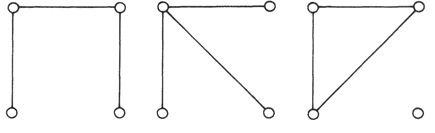The preceding discussion illustrates the following celebrated result from the field of combinatorics. (Note: For a real number x, the number {x} denotes the smallest integer greater than or equal to x.)

The Pigeonhole Principle
Let S be a finite set consisting of n elements, and let S1, S2, . . . , Sk be a partition of S into k subsets. Then at least one subset Si, 1 ≤ i ≤ k, contains at least {n/k} elements.

Hence, if there are three equivalence classes of (4, 3) graphs (that is, k = 3), and we have four (4, 3) graphs (that is, n = 4), then there must be at least {4/3} = 2 graphs in the same equivalence class.

### 2.3 Connected Graphs

Probably the most important class of graphs is the class of connected graphs. In this section we discuss connected graphs together with some related concepts.

Let G be a graph. A graph H is a subgraph of G if V(H) ⊆ V(G) and E(H) ⊆ E(G). If a graph F is isomorphic to a subgraph H of G, then F is also called a subgraph of G. Figure 2.11 shows a graph G and a subgraph H.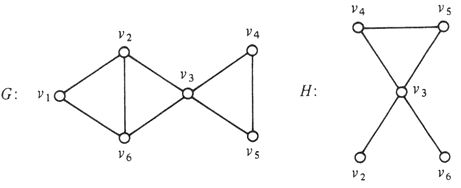Let u and v be vertices of a graph G. A u−v walk in G is an alternating sequence of vertices and edges of G, beginning with u and ending with v, such that every edge joins the vertices immediately preceding it and following it. For example, v3, v3v2, v2, v2v6, v6. v6v3, v3, v3v4, v4, v4v5, v5, v5v4, v4 is a v3−v4 walk in the graph G of Figure 2.11. Observe that the edge v4v5 appears twice in this walk. We need only list the vertices in a walk, for the edges are then obvious. The walk just described can therefore be expressed more simply as v3, v2, v6, v3, v4, v5, v4.

A u−v trail in a graph is a u−v walk which does not repeat any edge. The v3−v4 walk described above is not a v3−v4 trail; however, v3, v2, v6, v3, v4 is a v3−v4 trail in the graph G of Figure 2.11. A u−v path is a u−v walk (or u−v trail) which does not repeat any vertex. Again, in the graph G of Figure 2.11, v3, v5, v4 is a v3−v4 path.

Two vertices u and v in a graph G are connected if u = v, or if u ≠ v and a u−v path exists in G. A graph G is connected if every two vertices of G are connected; otherwise, G is disconnected.

A connected subgraph H of a graph G is called a component of G if H is not contained in any connected subgraph of G having more vertices or edges than H. For example, the graph of Figure 2.12 has four components. If a graph has only one component, the graph is connected.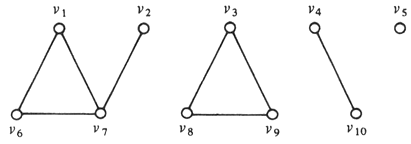A u−v trail in which u = v and which contains at least three edges is called a circuit. In other words, a circuit must end at the same vertex with which it began. A circuit which does not repeat any vertices (except the first and last) is called a cycle. For example, in the graph G of Figure 2.13, v1 v2, v3, v5, v2, v6, v1 is a circuit but is not a cycle, while v2, v4, v3, v5, v2 is a cycle (as well as a circuit).

By definition, a trail is an alternating sequence of vertices and edges, although we have agreed to represent a trail by a sequence of vertices. The sets of vertices and of edges determined by a trail produce a subgraph; it is also customary to refer to this subgraph as a trail. For example, v1, v2, v5, v3, v2, v6 is a trail in the graph G of Figure 2.13. If we define the subgraph H of G by V(H) = {v1, v2, v5, v3, v6} and E(H) = {v1v2, v2v5, v5v3, v3v2, v2v6}, then H is also called a trail in G. More generally, it is customary to consider the subgraph consisting of the vertices and edges of a trail, path, circuit, or cycle as the respective trail, path, circuit, or cycle.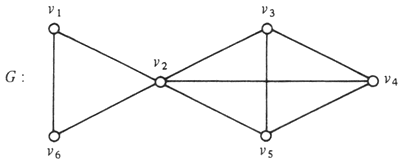### 2.4 Cut-Vertices and Bridges

We now introduce a class of vertices and a class of edges which are important and similar in many ways.

If e is an edge of a graph G, then G − e is the subgraph of G possessing the same vertex set as G and having all edges of G except e. If v is a vertex of a graph G containing at least two vertices, then G − v is the subgraph of G whose vertex set consists of all vertices of G except v and whose edge set consists of all edges of G except those incident with v. Figure 2.15 illustrates these concepts.

A vertex v in a connected graph G is called a cut-vertex if G − v is disconnected. The vertex v3 of the graph G in Figure 2.15 is a cut-vertex; however, no other vertex of that graph is a cut-vertex.

Now we consider the related concept for edges. An edge e in a connected graph G is called a bridge if G − e is disconnected. The edge e4 of the graph G in Figure 2.15 is a bridge, but no other edge of that graph is a bridge.If v is a cut-vertex of a connected graph G, then G − v contains two or more components. However, if e is a bridge of G, then G − e has exactly two components. The following theorem shows which edges of a graph are bridges.

Theorem 2.5
Let G be a connected graph. An edge e of G is a bridge of G if and only if e does not lie on any cycle of G.

Proof
Let e be a bridge of G. We prove the desired result by a contradiction argument. Suppose e = uv and e does lie on a cycle, say C: u, v, w, . . . , x, u (that is, w follows v on C and x precedes u). The graph G − e contains a u−v path, namely u, x, . . . , w, v, so that u is connected to v. We now show that G − e is connected.

Let u, and v1 be any two vertices of G − e; we show that G − e contains a u1−v1 path. Since G is connected, there is a u1−v1 path P in G. If the edge e does not lie on P. then P is also a path in G − e and u, is connected to v1 in G − e. Suppose the edge e lies on P. Then the path P can be expressed as u1, . . . , u, v, . . . , v1, or u1, . . . , v, u, . . . , v1. In the first case, u1 is connected to u and v is connected to v1 in G − e, and in the second case, u1 is connected to v and u is connected to v1. We have already observed that u is connected to v in G − e. By Exercise 2.44, the relation “is connected to” is an equivalence relation on V(G − e); hence the relation is transitive, implying that u1 is connected to v1. Therefore, if e belongs to a cycle, then G − e is connected and e is not a bridge. This produces the desired contradiction.

Conversely, suppose e = uv is an edge which lies on no cycle of G. Again, we give a proof by contradiction. Assume that e is not a bridge. Then G − e is connected, and thus, there is a u−v path P in G − e. However, P together with e produces a cycle in G containing e. Hence, we have a contradiction. ▋